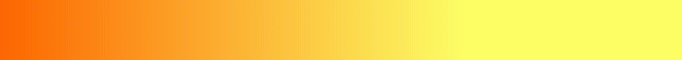The Beauty of Math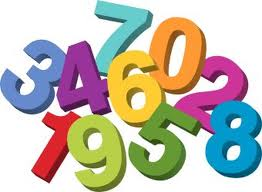1 x 8 + 1 = 9
12 x 8 + 2 = 98
123 x 8 + 3 = 987
1234 x 8 + 4 = 9876
12345 x 8 + 5 = 98765
123456 x 8 + 6 = 987654
1234567 x 8 + 7 = 9876543
12345678 x 8 + 8 = 98765432
123456789 x 8 + 9 = 987654321

1 x 9 + 2 = 11
12 x 9 + 3 = 111
123 x 9 + 4 = 1111
1234 x 9 + 5 = 11111
12345 x 9 + 6 = 111111
123456 x 9 + 7 = 1111111
1234567 x 9 + 8 = 11111111
12345678 x 9 + 9 = 111111111
123456789 x 9 +10 = 1111111111

9 x 9 + 7 = 88
98 x 9 + 6 = 888
987 x 9 + 5 = 8888
9876 x 9 + 4 = 88888
98765 x 9 + 3 = 888888
987654 x 9 + 2 = 8888888
98765 43 x 9 + 1 = 88888888
98765432 x 9 + 0 = 88888888

Brilliant!

Now, look at this symmetry.

1 x 1 = 1
11 x 11 = 121
111 x 111 = 12321
1111 x 1111 = 1234321
11111 x 11111 = 123454321
111111 x 111111 = 12345654321
1111111 x 1111111 = 1234567654321
11111111 x 11111111 = 123456787654321
111111111 x 111111111 = 12345678987654321

Now, from a strictly mathematical viewpoint, take a look at the much used expression "100%."

Ever been in a situation where someone asks you to give a 100%?

What equals 100%?

And what does it mean to give MORE than 100%?

Ever wondered what people mean when they say they are giving more than 100%?

Here's a formula that might help answer these questions.

When the letters A B C D E F G H I J K L M N O P Q R S T U V W X Y Z, in order, are represented numerically as 1 2 3 4 5 6 7 8 9 10 11 12 13 14 15 16 17 18 19 20 21 22 23 24 25 26, then

K-N-O-W-L-E-D-G-E = 11+14+15+23+12+5+4+7+5 = 96%;

H-A-R-D-W-O-R-K = 8+1+18+4+23+15+18+11 = 98%;

A-T-T-I-T-U-D-E = 1+20+20+9+20+21+4+5 = 100%;

and L-O-V-E-O-F-G-O-D = 12+15+22+5+15+6+7+15+4 = 101%.

We can conclude, with mathematical certainty, that while KNOWLEDGE and HARD WORK will get us close, and ATTITUDE will actually get us there, it's the LOVE OF GOD that will put us over the top! -- See Using Figures To Figure IT. Or watch this.The Secret Code, Number 9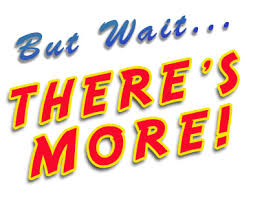The Secret Behind Numbers 369, The Tesla Code

Math Riddle: Why was 6 afraid of 7? Answer:Life Path Numerology

Birthday Numerology

The Beauty of Math

The 'Beauties' of Math

A kid's answer to, "Find X!"See What Makes People Smart.Now you know!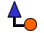More Web sites by
KhalsaWebMasters.com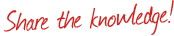®